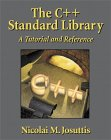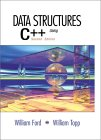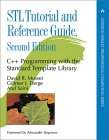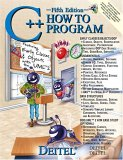# C++ STL (Standard Template Library) Tutorial and Examples

C++ STL Tutorial Contents:

Contents:

Related YoLinux Tutorials:

STL Description:

The Standard Template Libraries (STL's) are a set of C++ template classes to provide common programming data structures and functions such as doubly linked lists (list), paired arrays (map), expandable arrays (vector), large string storage and manipulation (rope), etc. The STL library is available from the STL home page. This is also your best detailed reference for all of the STL class functions available.

Also see our C++ Templates tutorial

STL can be categorized into the following groupings:

• Container classes:
• Sequences:
• Associative Containers:
• set (duplicate data not allowed in set), multiset (duplication allowed): Collection of ordered data in a balanced binary tree structure. Fast search.
• map (unique keys), multimap (duplicate keys allowed): Associative key-value pair held in balanced binary tree structure.
• stack LIFO
• queue FIFO
• priority_queue returns element with highest priority.
• String:
• string: Character strings and manipulation
• rope: String storage and manipulation
• bitset: Contains a more intuitive method of storing and manipulating bits.
• Operations/Utilities:
• iterator: (examples in this tutorial) STL class to represent position in an STL container. An iterator is declared to be associated with a single container class type.
• algorithm: Routines to find, count, sort, search, ... elements in container classes
• auto_ptr: Class to manage memory pointers and avoid memory leaks.

STL vector:

vector: Dynamic array of variables, struct or objects. Insert data at the end.

Simple example of storing STL strings in a vector. This example shows three methods of accessing the data within the vector:

```#include <iostream>
#include <vector>
#include <string>

using namespace std;

main()
{
vector<string> SS;

SS.push_back("The number is 10");
SS.push_back("The number is 20");
SS.push_back("The number is 30");

cout << "Loop by index:" << endl;

int ii;
for(ii=0; ii < SS.size(); ii++)
{
cout << SS[ii] << endl;
}

cout << endl << "Constant Iterator:" << endl;

vector<string>::const_iterator cii;
for(cii=SS.begin(); cii!=SS.end(); cii++)
{
cout << *cii << endl;
}

cout << endl << "Reverse Iterator:" << endl;

vector<string>::reverse_iterator rii;
for(rii=SS.rbegin(); rii!=SS.rend(); ++rii)
{
cout << *rii << endl;
}

cout << endl << "Sample Output:" << endl;

cout << SS.size() << endl;
cout << SS << endl;

swap(SS, SS);
cout << SS << endl;
}
```

Compile: g++ exampleVector.cpp

Run: ./a.out

Output:

```Loop by index:
The number is 10
The number is 20
The number is 30

Constant Iterator:
The number is 10
The number is 20
The number is 30

Reverse Iterator:
The number is 30
The number is 20
The number is 10

Sample Output:
3
The number is 30
The number is 10
```

[Potential Pitfall]: Note that the iterator is compared to the end of the vector with "!=". Do not use "<" as this is not a valid comparison and may or may not work. The use of "!=" will always work.

Two / Three / Multi Dimensioned arrays using vector:

A two dimensional array is a vector of vectors. The vector contructor can initialize the length of the array and set the initial value.

Example of a vector of vectors to represent a two dimensional array:
```#include <iostream>
#include <vector>

using namespace std;

main()
{
// Declare size of two dimensional array and initialize.
vector< vector<int> > vI2Matrix(3, vector<int>(2,0));

vI2Matrix = 0;
vI2Matrix = 1;
vI2Matrix = 10;
vI2Matrix = 11;
vI2Matrix = 20;
vI2Matrix = 21;

cout << "Loop by index:" << endl;

int ii, jj;
for(ii=0; ii < 3; ii++)
{
for(jj=0; jj < 2; jj++)
{
cout << vI2Matrix[ii][jj] << endl;
}
}
}
```

Compile: g++ exampleVector2.cpp

Run: ./a.out

```Loop by index:
0
1
10
11
20
21
```

A three dimensional vector would be declared as:

```#include <iostream>
#include <vector>

using namespace std;

main()
{
// Vector length of 3 initialized to 0
vector<int> vI1Matrix(3,0);

// Vector length of 4 initialized to hold another
// vector vI1Matrix which has been initialized to 0
vector< vector<int> > vI2Matrix(4, vI1Matrix);

// Vector of length 5 containing two dimensional vectors
vector< vector< vector<int> > > vI3Matrix(5, vI2Matrix);

...
```

or declare all in one statement:

```#include <iostream>
#include <vector>

using namespace std;

main()
{
vector< vector< vector<int> > > vI3Matrix(2, vector< vector<int> > (3, vector<int>(4,0)) );

for(int kk=0; kk<4; kk++)
{
for(int jj=0; jj<3; jj++)
{
for(int ii=0; ii<2; ii++)
{
cout << vI3Matrix[ii][jj][kk] << endl;
}
}
}
}
```

Using an iterator:

Example of iterators used with a two dimensional vector.
```#include <iostream>
#include <vector>

using namespace std;

main()
{
vector< vector<int> > vI2Matrix;    // Declare two dimensional array
vector<int> A, B;
vector< vector<int> >::iterator iter_ii;
vector<int>::iterator                 iter_jj;

A.push_back(10);
A.push_back(20);
A.push_back(30);
B.push_back(100);
B.push_back(200);
B.push_back(300);

vI2Matrix.push_back(A);
vI2Matrix.push_back(B);

cout << endl << "Using Iterator:" << endl;

for(iter_ii=vI2Matrix.begin(); iter_ii!=vI2Matrix.end(); iter_ii++)
{
for(iter_jj=(*iter_ii).begin(); iter_jj!=(*iter_ii).end(); iter_jj++)
{
cout << *iter_jj << endl;
}
}
}
```

Compile: g++ exampleVector2.cpp

Run: ./a.out

```Using Iterator:
10
20
30
100
200
300
```

[Potential Pitfall]: Note that "end()" points to a position after the last element and thus can NOT be used to point to the last element.

```iter_jj = SS.end();
cout << *iter_jj << endl;
```

This will result in a "Segmentation fault" error.

Vector member functions:

#### Constructor/Declaration:

Method/operator Description
vector<T> v; Vector declaration of data type "T".
vector<T> v(size_type n); Declaration of vector containing type "T" and of size "n" (quantity).
vector<T> v(size_type n,const T& t); Declaration of vector containing type "T", of size "n" (quantity) containing value "t".
Declaration: vector(size_type n, const T& t)
vector<T> v(begin_iterator,end_iterator); Copy of Vector of data type "T" and range begin_iterator to end_iterator.
Declaration: template vector(InputIterator, InputIterator)

#### Size methods/operators:

Method/operator Description
empty() Returns bool (true/false). True if empty.
Declaration: bool empty() const
size() Number of elements of vector.
Declaration: size_type size() const
resize(n, t=T()) Adjust by adding or deleting elements of vector so that its size is "n".
Declaration: void resize(n, t = T())
capacity() Max number of elements of vector before reallocation.
Declaration: size_type capacity() const
reserve(size_t n) Max number of elements of vector set to "n" before reallocation.
Declaration: void reserve(size_t)
max_size() Max number of elements of vector possible.
Declaration: size_type max_size() const
Note: size_type is an unsigned integer.

#### Other methods/operators:

Method/operator Description
erase()
clear()
Erase all elements of vector.
Declaration: void clear()
erase(iterator)
erase(begin_iterator,end_iterator)
Erase element of vector. Returns iterator to next element.
Erase element range of vector. Returns iterator to next element.
Declarations:
• iterator erase(iterator pos)
• iterator erase(iterator first, iterator last)
=
Example: X=Y()
Assign/copy entire contents of one vector into another.
Declaration: vector& operator=(const vector&)
< Comparison of one vector to another.
Declaration: bool operator<(const vector&, const vector&)
== Returns bool. True if every element is equal.
Declaration: bool operator==(const vector&, const vector&)
at(index)
v[index]
Element of vector. Left and Right value assignment: v.at(i)=e; and e=v.at(i);
Declaration: reference operator[](size_type n)
front()
v
First element of vector. (Left and Right value assignment.)
Declaration: reference front()
back() Last element of vector. (Left and Right value assignment.)
Declaration: reference back()
push_back(const T& value) Add element to end of vector.
Declaration: void push_back(const T&)
pop_back() Remove element from end of vector.
Declaration: void pop_back()
assign(size_type n,const T& t) Assign first n elements a value "t".
assign(begin_iterator,end_iterator) Replace data in range defined by iterators.
Declaration:
insert(iterator, const T& t) Insert at element "iterator", element of value "t".
Declaration: iterator insert(iterator pos, const T& x)
insert(iterator pos, size_type n, const T& x) Starting before element "pos", insert first n elements of value "x".
Declaration: void insert(iterator pos, size_type n, const T& x)
insert(iterator pos, begin_iterator,end_iterator) Starting before element "pos", insert range begin_iterator to end_iterator.
Declaration: void insert(iterator pos, InputIterator f, InputIterator l)
swap(vector& v2) Swap contents of two vectors.
Declaration: void swap(vector&)

#### Iterator methods/operators:

Method/operator Description
begin() Return iterator to first element of vector.
Declaration: const_iterator begin() const
end() Return iterator to end of vector (not last element of vector but past last element)
Declaration: const_iterator end() const
rbegin() Return iterator to first element of vector (reverse order).
Declaration: const_reverse_iterator rbegin() const
rend() Return iterator to end of vector (not last element but past last element) (reverse order).
Declaration: const_reverse_iterator rend() const
++ Increment iterator.
-- Decrement iterator.

STL list:

list: Linked list of variables, struct or objects. Insert/remove anywhere.

Two examples are given:

1. The first STL list example is using a native data type (int)
2. The second for a list of objects (class instances)
They are used to show a simple example and a more complex real world application.

1) Storing native data types:

Lets start with a simple example of a program using STL for a linked list to store integers:

```// Standard Template Library example

#include <iostream>
#include <list>
using namespace std;

// Simple example uses type int

main()
{
list<int> L;
L.push_back(0);              // Insert a new element at the end
L.push_front(0);             // Insert a new element at the beginning
L.insert(++L.begin(),2);     // Insert "2" before position of first argument
// (Place before second argument)
L.push_back(5);
L.push_back(6);

list<int>::iterator i;

for(i=L.begin(); i != L.end(); ++i) cout << *i << " ";
cout << endl;
return 0;
}
```

Compile: g++ example1.cpp

Run: ./a.out

Output: 0 2 0 5 6

[Potential Pitfall]: In Red Hat Linux versions 7.x one could omit the "using namespace std;" statement. Use of this statement is good programming practice and is required in Red Hat 8.0 and later.

[Potential Pitfall]: Red Hat 8.0 and later requires the reference to "#include <iostream>". Red Hat versions 7.x used "#include <iostream.h>".

2) Storing objects:

The following example stores a class object in a doubly linked list. In order for a class to be stored in an STL container, it must have a default constructor, the class must be assignable, less than comparable and equality comparable.

Since we are storing class objects and we are not using defined built-in C++ types we have therefore included the following:

• To make this example more complete, a copy constructor has been included although the compiler will generate a member-wise one automatically if needed. This has the same functionality as the assignment operator (=).
• The assignment (=) operator must be specified so that sort routines can assign a new order to the members of the list.
• The "less than" (<) operator must be specified so that sort routines can determine if one class instance is "less than" another.
• The "equals to" (==) operator must be specified so that sort routines can determine if one class instance is "equals to" another.

```// Standard Template Library example using a class.

#include <iostream>
#include <list>
using namespace std;

// The List STL template requires overloading operators =, == and <.

class AAA
{
friend ostream &operator<<(ostream &, const AAA &);

public:
int x;
int y;
float z;

AAA();
AAA(const AAA &);
~AAA(){};
AAA &operator=(const AAA &rhs);
int operator==(const AAA &rhs) const;
int operator<(const AAA &rhs) const;
};

AAA::AAA()   // Constructor
{
x = 0;
y = 0;
z = 0;
}

AAA::AAA(const AAA &copyin)   // Copy constructor to handle pass by value.
{
x = copyin.x;
y = copyin.y;
z = copyin.z;
}

ostream &operator<<(ostream &output, const AAA &aaa)
{
output << aaa.x << ' ' << aaa.y << ' ' << aaa.z << endl;
return output;
}

AAA& AAA::operator=(const AAA &rhs)
{
this->x = rhs.x;
this->y = rhs.y;
this->z = rhs.z;
return *this;
}

int AAA::operator==(const AAA &rhs) const
{
if( this->x != rhs.x) return 0;
if( this->y != rhs.y) return 0;
if( this->z != rhs.z) return 0;
return 1;
}

// This function is required for built-in STL list functions like sort
int AAA::operator<(const AAA &rhs) const
{
if( this->x == rhs.x && this->y == rhs.y && this->z < rhs.z) return 1;
if( this->x == rhs.x && this->y < rhs.y) return 1;
if( this->x < rhs.x ) return 1;
return 0;
}

main()
{
list<AAA> L;
AAA Ablob ;

Ablob.x=7;
Ablob.y=2;
Ablob.z=4.2355;
L.push_back(Ablob);  // Insert a new element at the end

Ablob.x=5;
L.push_back(Ablob);  // Object passed by value. Uses default member-wise
// copy constructor
Ablob.z=3.2355;
L.push_back(Ablob);

Ablob.x=3;
Ablob.y=7;
Ablob.z=7.2355;
L.push_back(Ablob);

list<AAA>::iterator i;

cout << "Print x: " << endl;
for(i=L.begin(); i != L.end(); ++i) cout << (*i).x << " "; // print member
cout << endl << endl;

cout << "Print x, y, z: " << endl;
for(i=L.begin(); i != L.end(); ++i) cout << *i; // print with overloaded operator
cout << endl;

cout << "Sorted: " << endl;
L.sort();
for(i=L.begin(); i != L.end(); ++i) cout << *i; // print with overloaded operator
cout << endl;

cout << "Iterate in Reverse: " << endl;
list<AAA>::reverse_iterator ri;
for(ri=L.rbegin(); ri != L.rend(); ++ri) cout << *ri; // print with overloaded operator
cout << endl;

cout << "Remove where x=5: " << endl;
for(i=L.begin(); i != L.end(); )       // Don't increment iterator here
if( (*i).x == 5 ) i = L.erase(i);  // Returns iterator to the next item in the list
else ++i;                          // Increment iterator here
for(i=L.begin(); i != L.end(); ++i) cout << *i; // print with overloaded operator
cout << endl;

return 0;
}

```

Output:

```Print x:
7 5 5 3

Print x, y, z:
7 2 4.2355
5 2 4.2355
5 2 3.2355
3 7 7.2355

Sorted:
3 7 7.2355
5 2 3.2355
5 2 4.2355
7 2 4.2355

Iterate in Reverse:
7 2 4.2355
5 2 4.2355
5 2 3.2355
3 7 7.2355

Remove where x=5:
3 7 7.2355
7 2 4.2355
```

STL vector vs list function comparison:
Functionvectorlistdeques
constructoryesyesyes
destructoryesyesyes
empty()yesyesyes
size()yesyesyes
max_size()yesyesyes
resize()yesyesyes
capacity()yesnono
reserve()yesnono
erase()yesyesyes
clear()yesyesyes
operator=yesyesyes
operator<yesyesno
operator==yesyesno
operator[]yesnoyes
at()yesnoyes
front()yesyesyes
back()yesyesyes
push_back()yesyesyes
pop_back()yesyesyes
assign()yesyesyes
insert()yesyesyes
swap()yesyesyes
push_front()noyesyes
pop_front()noyesyes
merge()noyesno
remove()noyesno
remove_if()noyesno
reverse()noyesno
sort()noyesno
splice()noyesno
unique()noyesnoBooks:The C++ Standard Library: A Tutorial and Reference Nicolai M. Josuttis ISBN #0201379260, Addison Wesley Longman This book is the only book I have seen which covers string classes as implemented by current Linux distributions. It also offers a fairly complete coverage of the C++ Standard Template Library (STL). Good reference book.STL for C++ programmers Leen Ammeraal ISBN #0 471 97181 2, John Wiley & Sons Ltd. Short book which teaches C++ Standard Template Library (STL) by example. Not as great as a reference but is the best at introducing all the concepts necessary to grasp STL completely and good if you want to learn STL quickly. This book is easy to read and follow.Data Structures with C++ Using STL William Ford, Willaim Topp ISBN #0130858501, Prentice HallSTL Tutorial and Reference Guide: C++ Programming with the Standard Template Library David R. Musser, Gillmer J. Derge, Atul Saini ISBN #0201379236, Addison-Wesley PublicationsThe C++ Templates: The complete guide. David Vandevoorde, Nicolai Josuttis ISBN #0201734842, Addison Wesley Pub Co. Covers complex use of C++ Templates.C++ How to Program by Harvey M. Deitel, Paul J. Deitel ISBN #0131857576, Prentice Hall Fifth edition. The first edition of this book (and Professor Sheely at UTA) taught me to program C++. It is complete and covers all the nuances of the C++ language. It also has good code examples. Good for both learning and reference.Amazon.com Widgets